### Magnetic Effects of Electric Current - Test Papers

CBSE Test Paper-01

Chapter 13 Magnetic Effects of Electric Current

1. The phenomenon of electromagnetic induction is (1)
1. the process of rotating a coil at an electric motor
2. the process of charging a body
3. the process of generating magnetic field due to a current passing through a coil
4. producing induced current in a coil due to relative motion between a magnet and the coil
2. When a coil carries current in an anti clockwise direction what pole does it create? (1)
1. South pole
2. Zero pole
3. North pole
4.  both South pole and North pole
3. Match the following with correct response. (1)
 (1) Direction of induced current produced by motion of conductor in a magnetic field is given by (A) Fleming's left hand rule (2) Direction of force acting on a current carrying conductor kept in a magnetic field is given by (B) Earthing (3) Production of electricity from magnetism (C) Electromagnetic induction (4) High powered electrical appliances are connected to the earth (D) Fleming's right hand rule
1. 1-C, 2-B, 3-D, 4-A
2. 1-D, 2-A, 3-C, 4-B
3. 1-A, 2-C, 3-B, 4-D
4. 1-B, 2-D, 3-A, 4-C
4. Which of the following involves electro magnetic induction? (1)
1.  A magnetic field exerts a force on a current- carrying wire
2. An electric current produces a magnetic field.
3. A rod is charged with electricity
4. The relative motion between a magnet and a coil produces an electric current.
5. The fuse wire should have (1)
1. Low resistance, High melting point
2. Low resistance, Low melting point
3. High resistance, High melting point
4. High resistance, Low melting point
6. What does the divergence of magnetic field lines near the ends of a current carrying straight solenoid indicate? (1)
7. Identify the poles of a magnet in the figure. (1)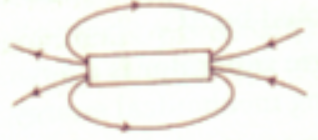8. Why is an alternating current considered to be advantageous over direct current for long range transmission of electric energy? (1)
9. Identify the region, where the magnetic field around a current carrying solenoid is uniform. (1)
1. Two circular coils P and Q are kept close to each other, of which, coil P carries a current. Will some current be induced in coil Q if coil P is moved towards Q ? Give a reason for your answer and name the phenomenon involved.
2. What happens, if coil P is moved away from Q?
3. State few methods of inducing current in a coil. (3)
10. Compare the permanent magnet and an electromagnet. (3)
11. A current carrying conductor is placed perpendicular to the uniform magnetic field. What happens to displacement of the conductor if
(i) Amount of current increases
(ii) If horse shoe magnet is replaced by a weak horse shoe magnet. (3)
12. A circular metallic loop is kept above the wire AB as shown below: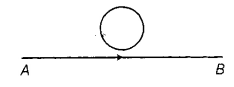What is the direction of induced current produced in the loop, if the current flowing in the straight wire
1. is steady, i.e. does not vary?
2. is increasing in magnitude?
13. What is the pattern of magnetic field due to a circular coil carrying current? (5)
14. Draw an appropriate schematic diagram showing common domestic circuits and discuss the importance of fuse. Why is it that a burnt out fuse should be replaced by another fuse of identical rating? (5)

CBSE Test Paper-01
Chapter 13 Magnetic Effects of Electric Current

1. producing induced current in a coil due to relative motion between a magnet and the coil
Explanation: When a straight coil and a magnet are moved relative to each other, a current is induced in the coil. This phenomenon is known as electromagnetic induction.
1. North pole
Explanation: According to clock rule if we look at the face of the coil which carries current in the clock wise direction then the face is called as south pole looking from the other side of coil we find that anti clock wise current flows that is called as north pole.
1. 1-D, 2-A, 3-C, 4-B
Explanation:
1. The direction of induced current in a straight conductor is given by Fleming’s right hand rule.
2. It states that if we stretch the thumb, forefinger and the middle finger of right hand at right angles to one another in such a way that the forefinger points in the direction of magnetic field.
3. Then, thumb gives the direction of motion of conductor (force), forefinger indicates direction of magnetic field and the middle finger points the direction of induced current.
4. The direction of force which acts on the current-carrying conductor placed in a magnetic field is given by Fleming’s left hand rule. It states that if the forefinger, thumb and middle finger of left hand are stretched mutually perpendicular and the forefinger points along the direction of external magnetic field, middle finger indicates the direction of current, then thumb points along the direction of force acting on the conductor.
5. Electromagnetic induction is the process of generating electric current with a magnetic field. It occurs whenever a magnetic field and an electric conductor, such as a coil of wire, move relative to one another.
6. High powered electrical appliances are connected to the earth by Earthing wire.
1. The relative motion between a magnet and a coil produces an electric current.
Explanation: When a straight coil and a magnet are moved relative to each other, a current is induced in the coil. This phenomenon is known as electromagnetic induction.
1. High resistance, Low melting point
Explanation: A fuse wire has high resistance and low melting point so that it will melt if a current of large magnitude passes through the wire.
1. The divergence of magnetic field lines indicates the decrease in strength of the magnetic field near the ends of the solenoid.
2. The poles of a magnet are marked in the figure as we know that out side magnet field lines moves N$\to$S and inside the Magnet field lines moves S$\to$N .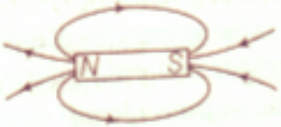3. Alternating current can be transmitted without much loss of electric energy to the distant places. So AC is considered to be advantageous over DC.
4. The magnetic field is uniform inside the magnet.
1. When coil P is moved towards Q, then current will be induced in coil Q because the coil P will carry a magnetic field around it, so when it is moved towards coil Q it increases the magnetic flux around it, hence, current is induced. This phenomenon is called electromagnetic induction.
2. If P is moved away from Q, then the magnetic flux around Q will decrease and a current will be induced but in the opposite direction.
3. Some of the methods of inducing current in the coil are as below:
1. Moving a magnet away or towards the coil.
2. Moving a coil away or towards a magnet.
3. Rotating a coil within a magnetic field.
4. By changing the magnitude of current.
5.  Permanent Magnet Electromagnet (i) Very strong electromagnets cannot be produced. (i) Very strong electromagnets can be produced. (ii) Its strength is fixed. (ii) Strength can be changed by changing the current through coil. (iii) Polarities are fixed. (iii) Polarities can be reversed by changing the direction of the current. (iv) Cannot be immediately demagnetized. (iv) Can be demagnetized immediately by stopping the current in the coil. (v) Example of permanent magnet is a Bar Magnet (v) Example of a temporary magnet is solenoid wounded across a nail and connected to a battery.
6. The displacement of the conductor
(i) will increase on increasing the current
(ii) Will decrease on using a weak horse shoe magnet.
1. The constant current flowing in the straight wire produces a constant magnetic field. Hence, no induced current is produced in the loop.
2. Since current in the straight wire is changing, hence, induced current will be produced in clockwise direction.
7. Magnetic field due to circular conductor: When a current is passed through a circular coil in clockwise direction, the magnetic field will be as shown in figure.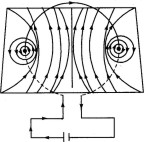Here the current is passing clockwise when looked from front side. At the points where the conductor passes through horizontal plane, the lines of force are almost circular, their direction being given by right-hand grip rule. Near the central region, the lines are quite straight and at right angles to the plane of the coil.
Further, it is clear from the figure that all the lines enter at the nearer face and leave at the farther face. Evidently, the lines of force due to the current flowing in circular loop closely resemble those produced by a magnetized circular disc of steel of same boundary as that of the coil, so that one face of it is north and other a south pole. The polarity of any face of coil can be determined by remembering a simple rule known as clock rule. If the current round any face of the coil flows in an anticlockwise direction it behaves like a north pole and if the current is in clockwise direction, the face acts as a south pole.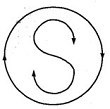Clockwise current behaves like south pole.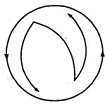Anti-clockwise current behaves like north pole.
8.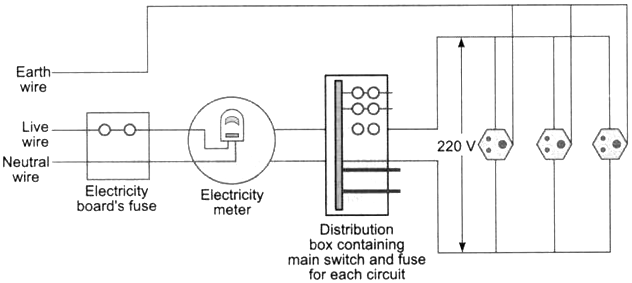A fuse in a circuit prevents damage to the appliances and the circuit due to overloading. Otherwise, the appliances or the circuit may be damaged.
When current in the circuit exceeds the value of fuse rating, the fuse wire burns due to overloading. This causes a gap in the circuit and the current stops flowing in the circuit.
This is done due to the reason so that the circuit or the appliances to be connected in the circuit continue functioning without any damage in future.